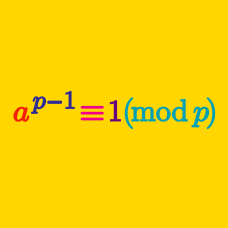Number Theory

# Basic Applications of Modular Arithmetic: Level 3 Challenges

Find the remainder when $3^{247}$ is divided by $17$.

$\Large 1+\dfrac{1}{2} + \dfrac{1}{3} + \ldots + \dfrac{1}{23} = \dfrac{a}{23!}$

Find the remainder when $a$ is divided by 13.

###### Try this set RMO Practice Problems

Find the last three digits of the number

$3 \times 7 \times 11 \times 15 \times \cdots \times 2003.$

Find the smallest positive integer $N$ such that $13^N \equiv 1 \pmod{2013}$.


Details and Assumptions:

• You may choose to refer to the modulo arithmetic notation.
• 0 is not a positive integer.

Find the GCD of $(19! + 19, 20! + 19).$


Note: GCD stands for the greatest common divisor.

×

Problem Loading...

Note Loading...

Set Loading...